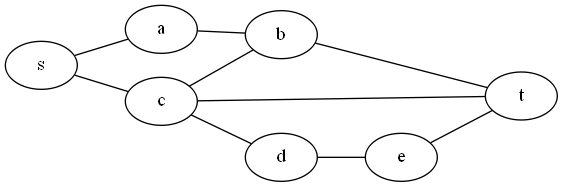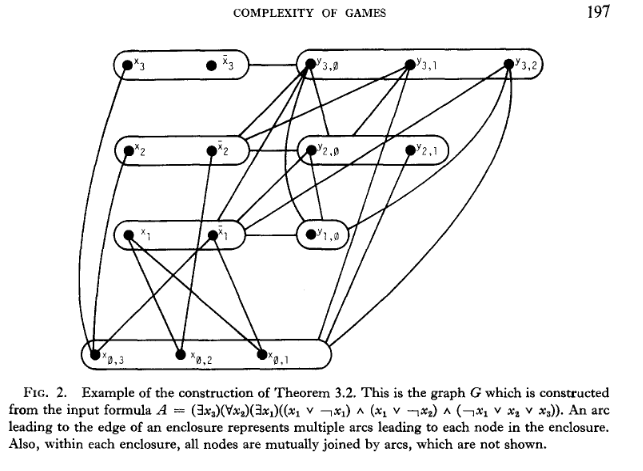# Generalized Kayles

I hadn’t heard of this game before encountering this problem.  It sort of feels a lot like Nim to me.

The problem: Generalized Kayles.  This is problem GP3 in the appendix.

The description: Given a graph G=(V,E). Players take turns removing vertices and all vertices adjacent to it from the graph.  The first player to have no vertices left loses.

Example: Here’s a graph:Suppose player 1 chooses vertex c.  Then we also remove s,b, d, and t.  All that is left are vertices a and e.  Whichever vertex player 2 chooses, player 1 can choose the other one, and win.

Reduction: This one is again by Schaefer, and again uses the same problem which is either Sequential Truth Assignment or QBF, depending on how you look at it.

Just like last time, we’re given a formula: (∃ x1) (∀ x2) (∃ x3) … (∃ xn) (A1 ∧ A2 ∧ … Am), where each Ai is a disjunction of literals.  We’ll further assume n is odd (so the last quantifier is ∃).  The graph is built as follows:

• Each clause k in the formula gets a vertex x0,k.  These vertices are all connected to each other.
• Each variable xi in the formula gets two vertices: xi and ~xi, that have an edge between them. We also get y vertices: yi,j for all j 0 <= j < i
• We add an edge between xi and x0,k if xi appears as a literal in clause k.  Similarly, we add an edge between ~xi and x0,k if ~xi appears as a literal in clause k.
• Each yi,j vertex connects to all xk vertices where k <= i.  Each yi,j vertex also connects to all ya,b vertices where a < i and b < a.

Here’s a picture from the paper of what we get:One main point that comes out of this construction is that players need to take their first n moves playing the x (or ~x) vertices in order.  If you go out of order, the opponent can play a y vertex and remove everything.  If you play a y vertex, the opponent can play an x vertex (or an x0 vertex) and remove everything.

If the original formula was satisfiable, player 1 (who is the ∃ player) starts by choosing either x1 or ~x1.  No matter which of x2 or ~x2 is chosen by the opponent (the ∀ player), player3 will have a way to set x3 to keep the formula satisfiable.  This process continues for all variables.  Once player 1 plays the last variable (xn or ~xn), all vertices have been removed from the graph- most importantly, all of the x0 vertices have been removed, because there is an edge from each one to each variable whose setting would satisfy the clause.  Thus, player 2 has no place to play, and player 1 wins.

If the formula is not satisfiable, then after taking turns choosing xi or ~xi for all i, there is still some x0 vertex in the graph (corresponding to a clause not satisfied by the variable choices made).  Player 2 can select that vertex, removing all x0 vertices from the graph, and will win.

Difficulty: 7.  I can see what the “jobs” of each vertex are: The xi  set the truth values of a variable, the x0 ensure that each clause is satisfied by a variable setting, and the y vertices are there to force players to choose the xi vertices in order.  I don’t think I could have come up with this though.